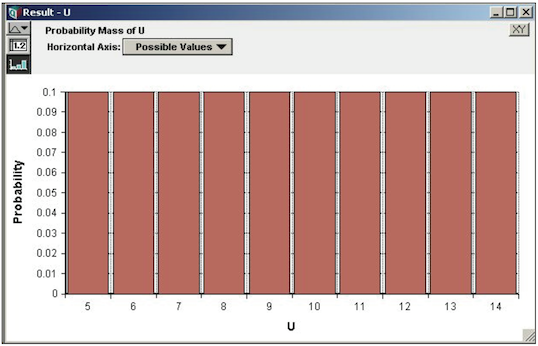# Uniform distribution

(diff) ← Older revision | Latest revision (diff) | Newer revision → (diff)

Release: 4.6  •  5.0  •  5.1  •  5.2  •  5.3  •  5.4  •  6.0  •  6.1  •  6.2  •  6.3  •  6.4

The uniform distribution assigns an equal probability to all outcomes between a lower bound, «min» and an upper bound «max». There are two variants of the uniform distribution -- the continuous uniform and the discrete (or integer) uniform. In the continuous uniform distribution, all real numbers between the bounds are equally likely. In the discrete uniform, all integers between the two bounds are equally likely.

If you know nothing about the uncertain quantity other than its bounds, a uniform distribution the bounds is appealing. However, situations in which this is truly appropriate are rare. Usually, you know that one end or the middle of the range is more likely than the rest — that is, the quantity has a mode. In such cases, a beta or triangular distribution is a better choice.

If you want a discrete uniform distribution over each value of an index I, use the ChanceDist distribution instead.

Continuous Uniform:

Uniform(5, 10) →Discrete (integer) Uniform:

Uniform(5, 14, Integer: True) →## Functions

### Uniform(min, max, integer, over)

The distribution function. Use this to define a quantify as being uniformly distributed between «min» and «max», or between 0 and 1 when «min» and «max» are omitted. Set «integer» to true for a discrete uniform over the integers between (and including) «min» and «max».

### DensUniform(x, min, max)

(New as a built-in function in Analytica 5.2 )

The probability density at «x» for a continuous uniform distribution. This is equal to

$\displaystyle{ p(x) = {1\over{max-min} } }$

when «min» <= «x» <=«max», and zero otherwise.

Use this one only with a continuous uniform.

### CumUniform(x, min, max, integer)

To use this function, add the Distribution Densities Library to your model.

The cumulative density function, describing the probability that the outcome is less than or equal to «x». This is equal to $\displaystyle{ F(x) = \left\{ \begin{array}{ll} 0 & \mbox{when } x \lt min \\ ( x - min ) / (max - min) & \mbox{when } min \leq x \leq max \\ 1 & \mbox{when } x\gt max \end{array}\right. }$

### CumUniformInv(p, min, max, integer)

To use this function, add the Distribution Densities Library to your model.

The inverse cumulative density function, also called the quantile function. Returns the value x such that the probability of the outcome being less than or equal to x is «p».

### Parameters

• «min», «max»: (Optional) The lower and upper bounds that define the distribution. When omitted, bounds of 0 and 1 are assumed. For an integer distribution, «min» and «max» are both included as possible outcomes (when these are integers, of course).
• «integer»: (Optional) A boolean. When omitted or false, the function specifies a continuous uniform distribution. When set to true, it specifies a discrete uniform distribution.
• «over»: (Optional) A list of indexes to independently sample over.
• «x»: The point were a density or probability is requested.
• «p»: The quantile probability requested.

## Examples

Uniform(5, 14, Integer: True) →Uniform(5, 10) →## Parameter Estimation

Suppose you have real-valued historic data in X, indexed by I, and you wish to estimate the bounds of the continuous uniform distribution. This is really just a matter of estimating the lower and upper bounds for the data, since the use of this distribution assumes a uniform distribution between those bounds. You can estimate the bounds as:

Xmin := Min(X, I) - 0.5*(Max(X, I) - Min(X, I))/Size(I);
Xmax := Max(X, I) + 0.5*(Max(X, I)- Min(X, I))/Size(I);

If you have discrete integer data in D indexed by I, you can estimate the parameters for the integer uniform distribution Uniform(Xmin, Xmax, integer: True) as:

Xmin := Floor(Min(D, I) - 0.5*(Max(D, I) - Min(D, I))/Size(I);
Xmax := Ceil(Max(D, I) + 0.5*(Max(D, I) - Min(D, I))/Size(I);